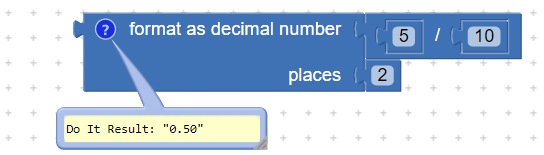# Decimal numer in calculator

how i write decimal number in label? I make a calculatorwith 4 actions for university classes in this programme and i don`t know how to add decimal number. If I divide 2 by 10, I get 5 on this calculator. Which blocks should i use and where it add?

Welcome to the community. You can use format as decimal block from maths drawer1 Like